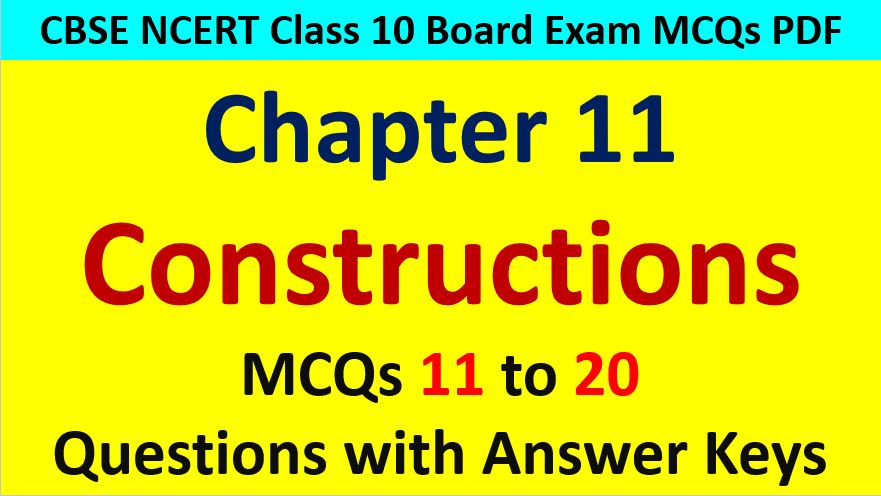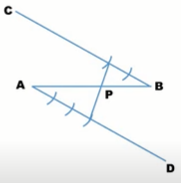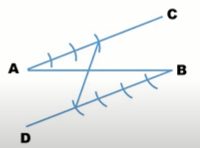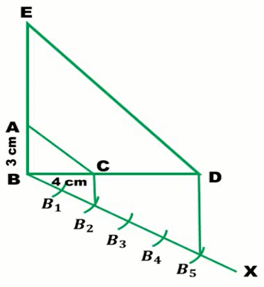Friday, October 22, 2021
Home > CBSE Class 10 > Constructions Class 10 Maths MCQ Questions with Answer Keys

# Constructions Class 10 Maths MCQ Questions with Answer KeysHi students, Welcome to AMBiPi (Amans Maths Blogs). In this article, you will get Constructions Class 10 Maths MCQ Questions with Answer Keys. You can download this PDF and save it in your mobile device or laptop etc.

Constructions MCQ Questions for Class 10 Question No 11:

To divide a line segment AB in the ratio 5 : 6, draw a ray AX such that ∠BAX is an acute angle, then draw a ray BY parallel too AX and the points A1, A2, A3, … and B1, B2, B3,… are located at equal distance on ray AX and BY respectively. Then, the points joined are

Option A : A5 and B6

Option B : A6 and B5

Option C : A4 and B5

Option D : A5 and B4

Option A : A5 and B6

Constructions MCQ Questions for Class 10 Question No 12:

To construct a triangle similar to a given ∆ABC, first draw a ray BX such that ∠CBX is an acute angle and X lies on the opposite side of A with respect to BC. Then, locate the points B1, B2, B3, … on BX at equal distance and next step is to join.

Option A : B10 to C

Option B : B3 to C

Option C : B7 to C

Option D : B4 to C

Option C : B7 to C

Constructions MCQ Questions for Class 10 Question No 13:

To construct a triangle similar to given triangle ABC with its sides 8/5 of the corresponding sides of ∆ABC draw a ray BX such that ∠CBX is an acute angle and X is on the opposite side of A with respect to BC. The minimum number of points to be located at equal distance on ray BX is

Option A : 5

Option B : 8

Option C : 13

Option D : 3

Option B : 8

Constructions MCQ Questions for Class 10 Question No 14:

To divide a line segment AB in the ratio p : q (p and q are positive integers), draw a ray AX so that ∠BAX is an acute angle and then mark point on ray AX at equal distance such that the minimum number of points on these points is

Option A : Greater of p and q

Option B : p + q

Option C : p + q – 1

Option D : pq

Option B : p + q

Constructions MCQ Questions for Class 10 Question No 15:

By geometrical construction, it is possible to divide a line segment in the ratio.

Option A : √3, 1/√3

Option B : √3, 1/√2

Option C : √3, √2

Option D : √3, √2/√3

Option A : √3, 1/√3

Constructions MCQ Questions for Class 10 Question No 16:

If a point P divides a line segment AB such that PB/AB = 3/7, then the ratio of AP : PB will be

Option A : 4 : 7

Option B : 7 : 4

Option C : 7 : 3

Option D : 4 : 3

Option D : 4 : 3

Constructions MCQ Questions for Class 10 Question No 17:

In the figure below, the ratio in which the point P divides the line segment AB isOption A : 3 : 2

Option B : 2 : 3

Option C : 2 : 1

Option D : 3 : 1

Option A : 3 : 2

Constructions MCQ Questions for Class 10 Question No 18:

In the figure below, the line segment AB is divided by the line in the ratio ofOption A : 3 : 2

Option B : 3 : 4

Option C : 4 : 3

Option D : 2 : 3

Option B : 3 : 4

Constructions MCQ Questions for Class 10 Question No 19:

The theorem, in which the division of a line segment is explain, is

Option A : Similarity criterion

Option B : Area Theorem

Option C : Basic Proportionality Theorem

Option D : Pythagoras Theorem,

Option A : 8

Constructions MCQ Questions for Class 10 Question No 20:

In the figure below, ∆ABC is constructed similar to ∆BDE. In ∆ABC = 90 degree, AB = 3 cm and BC = 4 cm. If the corresponding sides of ∆BDE is 5/2 times that of ∆ABC, then the length of the BD and DE respectively areOption A : 10 cm and 7.5 cm

Option B : 7.5 cm and 10 cm

Option C : 8 cm 12 cm

Option D : 12 cm, 8 cm